# Auxiliary Learning as an Asymmetric Bargaining Game

* equal contribution

 1Bar-Ilan University 2Aiola 3National University of Singapore 4NVIDIA Research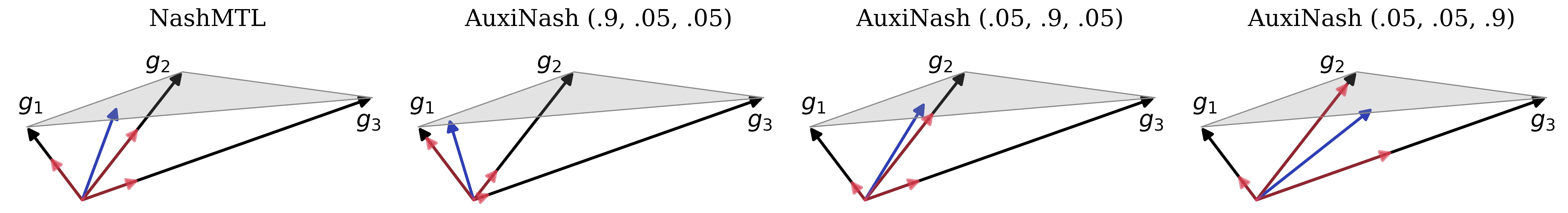Figure 1. Visualization of the update direction obtained by AuxiNash (Blue).

Auxiliary learning is an effective method for enhancing the generalization capabilities of trained models, particularly when dealing with small datasets. However, this approach may present several difficulties: (i) optimizing multiple objectives can be more challenging, and (ii) how to balance the auxiliary tasks to best assist the main task is unclear.

In this work, we propose a novel approach, named AuxiNash, for balancing tasks in auxiliary learning by formalizing the problem as generalized bargaining game with asymmetric task bargaining power. Furthermore, we describe an efficient procedure for learning the bargaining power of tasks based on their contribution to the performance of the main task and derive theoretical guarantees for its convergence. Finally, we evaluate AuxiNash on multiple multi-task benchmarks and find that it consistently outperforms competing methods.

## Auxiliary Learning

Auxiliary learning has a large potential to improve learning in the low data regime, but it gives rise to two main challenges: Defining the joint optimization problem and performing the optimization efficiently. (1) First, given a main task at hand, it is not clear which auxiliary tasks would benefit the main task and how tasks should be combined into a joint optimization objective. (2) Second, training with auxiliary tasks involves optimizing multiple objectives simultaneously; While training with multiple tasks can potentially improve performance via better generalization, it often underperforms compared to single-task models. Previous auxiliary learning research focused mainly on the first challenge: namely, weighting and combining auxiliary tasks. The second challenge, optimizing the main task in the presence of auxiliary tasks, has been less explored.

## Experiments

### Illustrative Example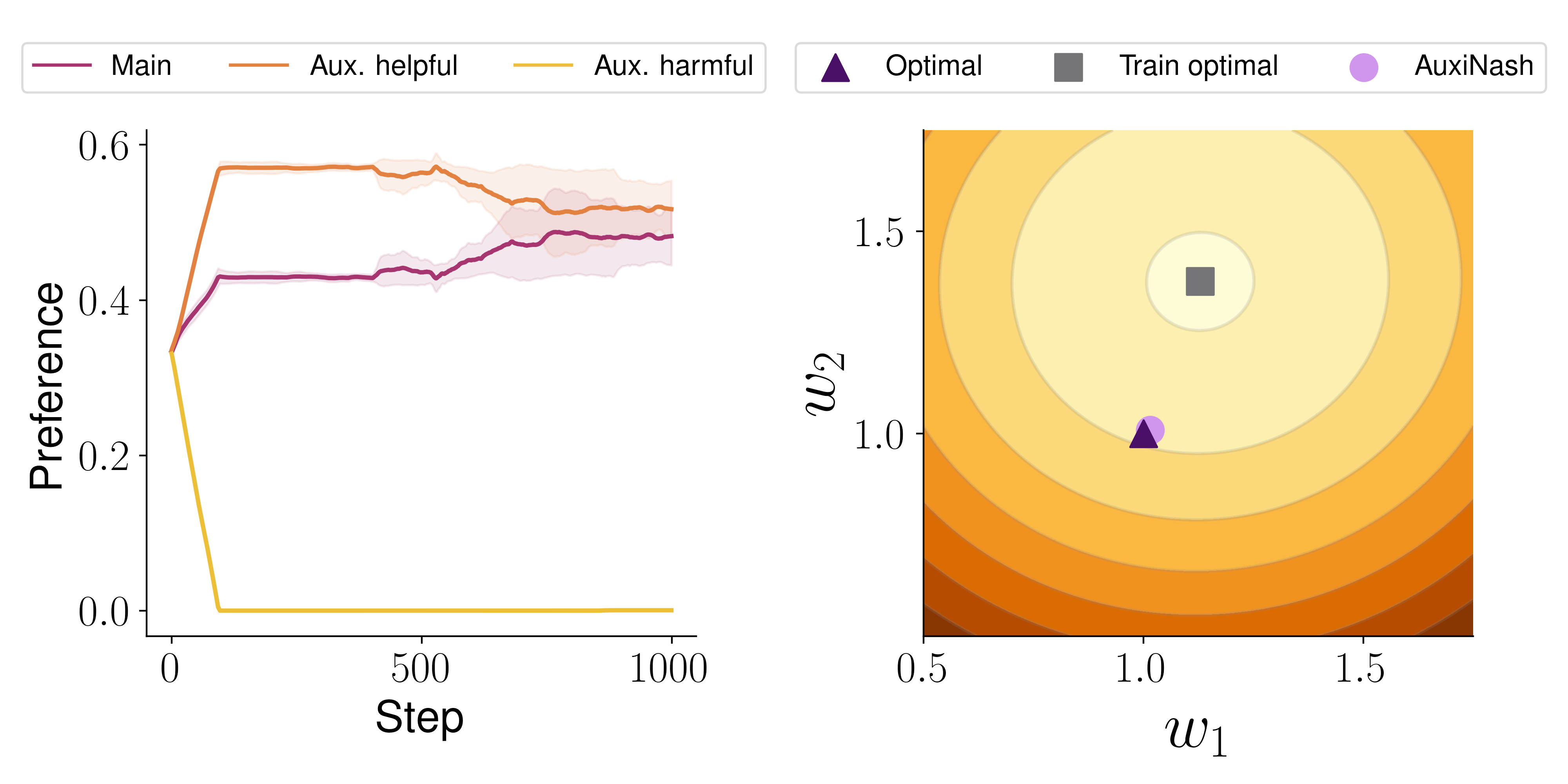Figure 2. Illustrative Example. A regression problem in $\mathbb{R}^2$ with two auxiliary tasks, one helpful and one harmful. We consider a regression problem with parameters $W^T=(w_1, w_2)\in\mathbb{R}^2$, fully shared among tasks. The optimal parameters for the main and helpful auxiliary tasks are $W^\star$, while the optimal parameters for the harmful auxiliary are $\tilde{W}\neq W^\star$. The main task is sampled from a Normal distribution $N({W^\star}^T x, \sigma_{\text{main}})$, with $\sigma_{\text{main}} > \sigma_{\text{h}}$ where $\sigma_{\text{h}}$ denotes the standard deviation for the noise of the helpful auxiliary. The change in the task preference throughout the optimization process is depicted in the left panel of Figure 2. AuxiNash identify the helpful tasks and fully ignore the harmful ones. In addition, Figure 2 right panel presents the main task's loss landscape, along with the optimal solution ($W^\star$, marked $\blacktriangle$), the optimal training set solution of the main task alone (${\blacksquare}$) and the solution obtained by AuxiNash (marked $\bullet$). While using the training data alone with no auxiliary information yields a solution that generalizes poorly, AuxiNash converges to a solution with large proximity to the optimal solution $W^\star$,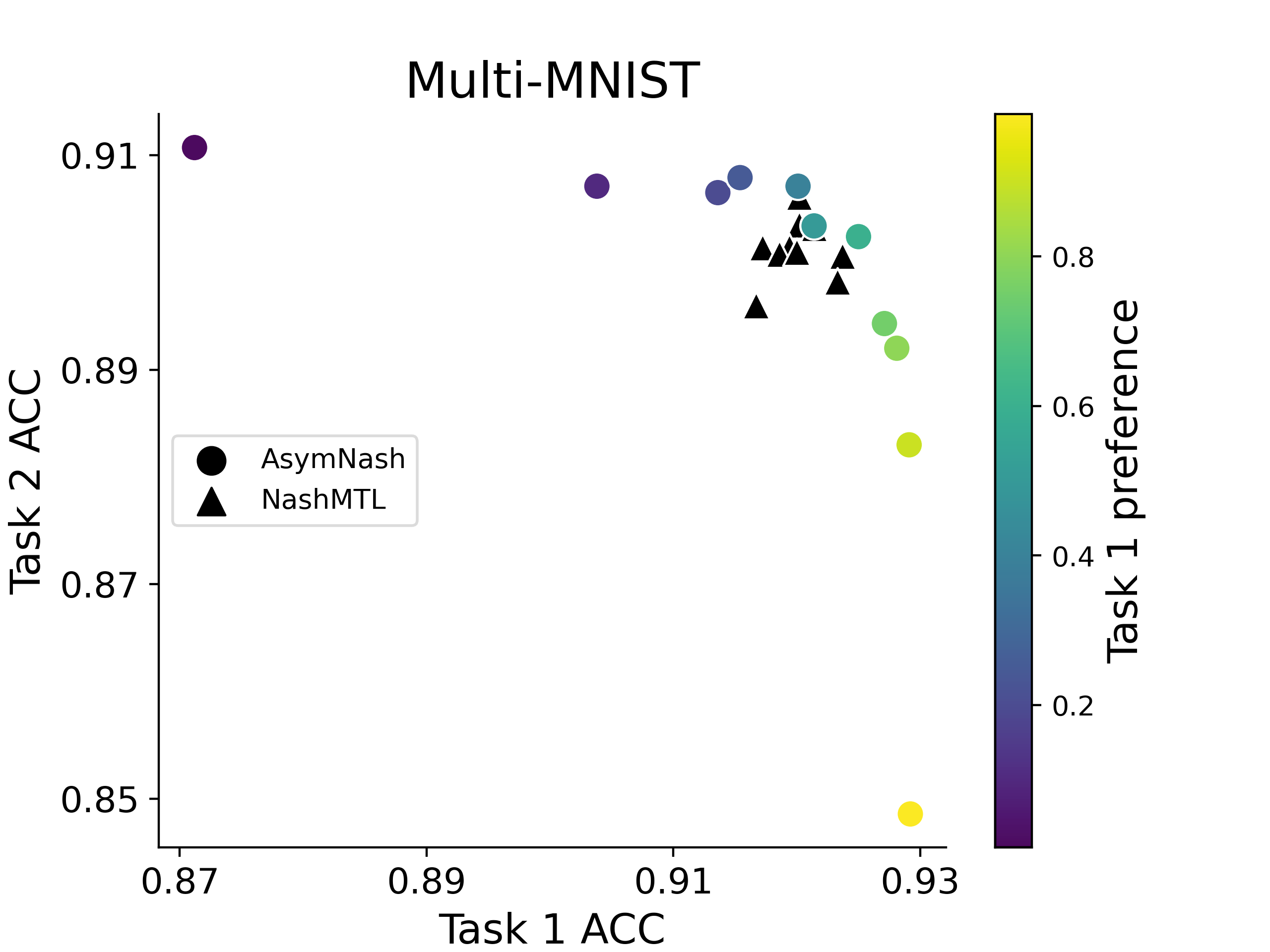Figure 3. Task Preferences. By varying the preference vector $p$, we show that AuxiNash can control the trade-off between tasks. Here, we show that controlling the preference vector can be used to steer the optimization outcome to different parts of the Pareto front, compared to the NashMTL baseline. We consider MTL setup with 2 image classification tasks and use the Multi-MNIST dataset. We run AuxiNash $11$ times with varying preference vector values $p$ and fix it throughout the training. For both tasks we report the classification accuracy. For Nash-MTL we run the experiments with different seed values. Figure 3 shows the results. AuxiNash reaches a diverse set of solutions across the Pareto front while Nash-MTL solutions are all relatively similar due to its symmetry property.

### Scene Understanding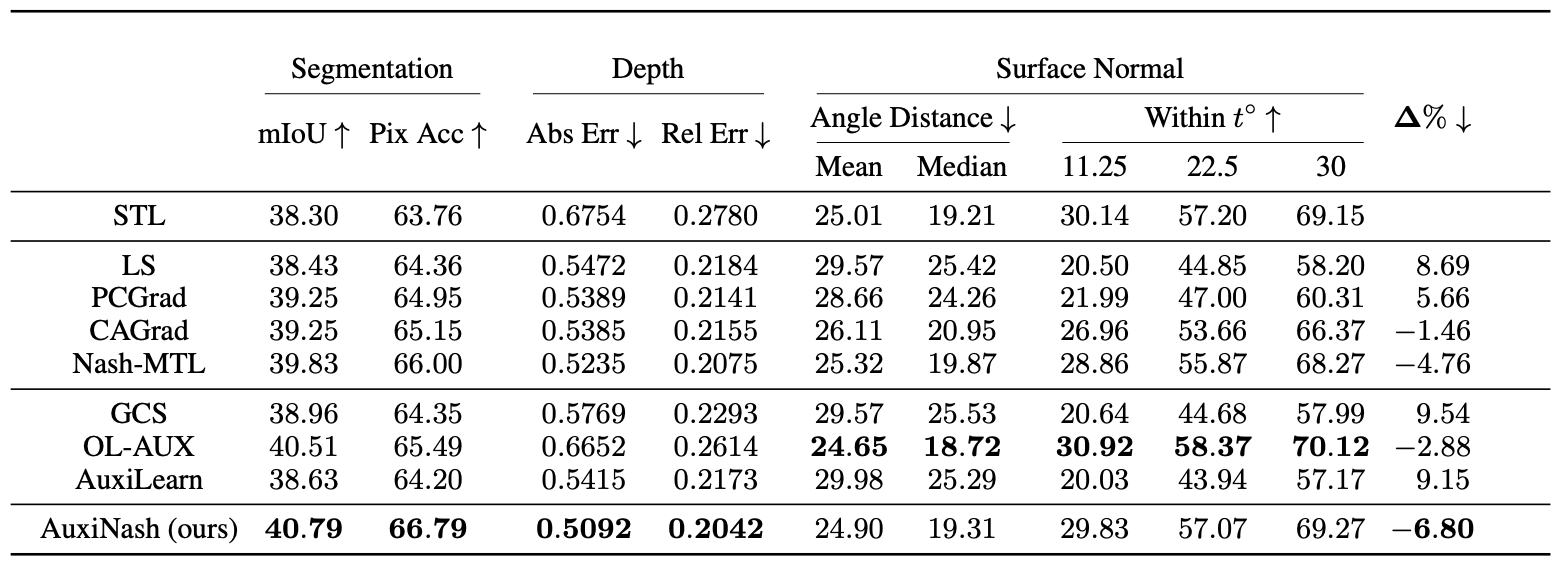Table 1. NYUv2. Test performance for three tasks: semantic segmentation, depth estimation, and surface normal. Here, we evaluate AuxiNash on Cityscapes and NYUv2 datasets. The indoor scene NYUv2 dataset contains 3 tasks: 13 classes semantic segmentation, depth estimation, and surface normal prediction. We also use the Cityscapes dataset with 3 tasks: 19-class semantic segmentation, disparity (inverse depth) estimation, and 10-class part segmentation. The results are presented in Table 1 and Table 2.
Observing the results, we can see our approach AuxiNash outperforms other approaches by a significant margin.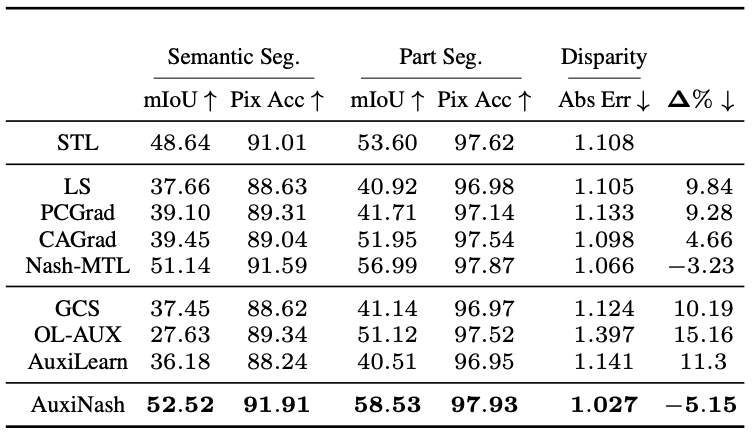Table 2. Cityscapes. Test performance for three tasks: 19-class semantic segmentation, 10-class part segmentation, and disparity.

### Semi Supervised Learning with SSL Auxiliaries

We evaluate AuxiNash on a Self-supervised Semi-supervised Learning setting. We use CIFAR-10 dataset to form 3 tasks. We set the supervised classification as the main task along with two self-supervised learning (SSL) tasks used as auxiliaries. For the supervised task we randomly allocate samples from the training set. We repeat this experiment twice with $5K$ and $10K$ labeled training examples.
The results are presented in Table 3. AuxiNash significantly outperforms most baselines.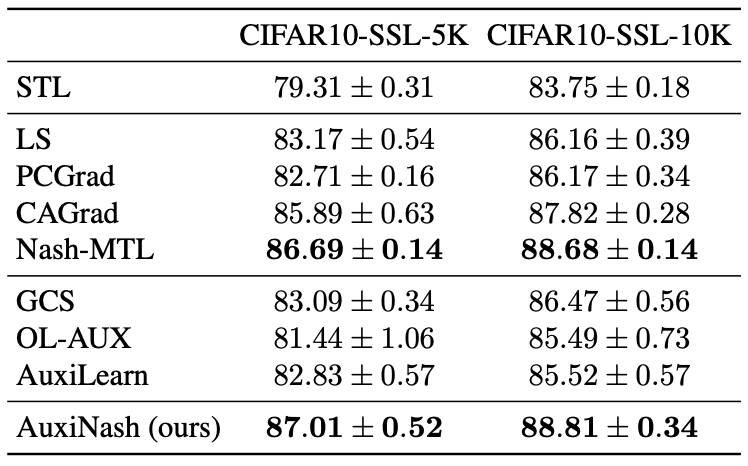Table 3. CIFAR10-SSL. Test performance for classification with a varying number of labeled data.

## Bibtex

@article{shamsian2023auxiliary, title={Auxiliary Learning as an Asymmetric Bargaining Game}, author={Shamsian, Aviv and Navon, Aviv and Glazer, Neta and Kawaguchi, Kenji and Chechik, Gal and Fetaya, Ethan}, journal={arXiv preprint arXiv:2301.13501}, year={2023} }